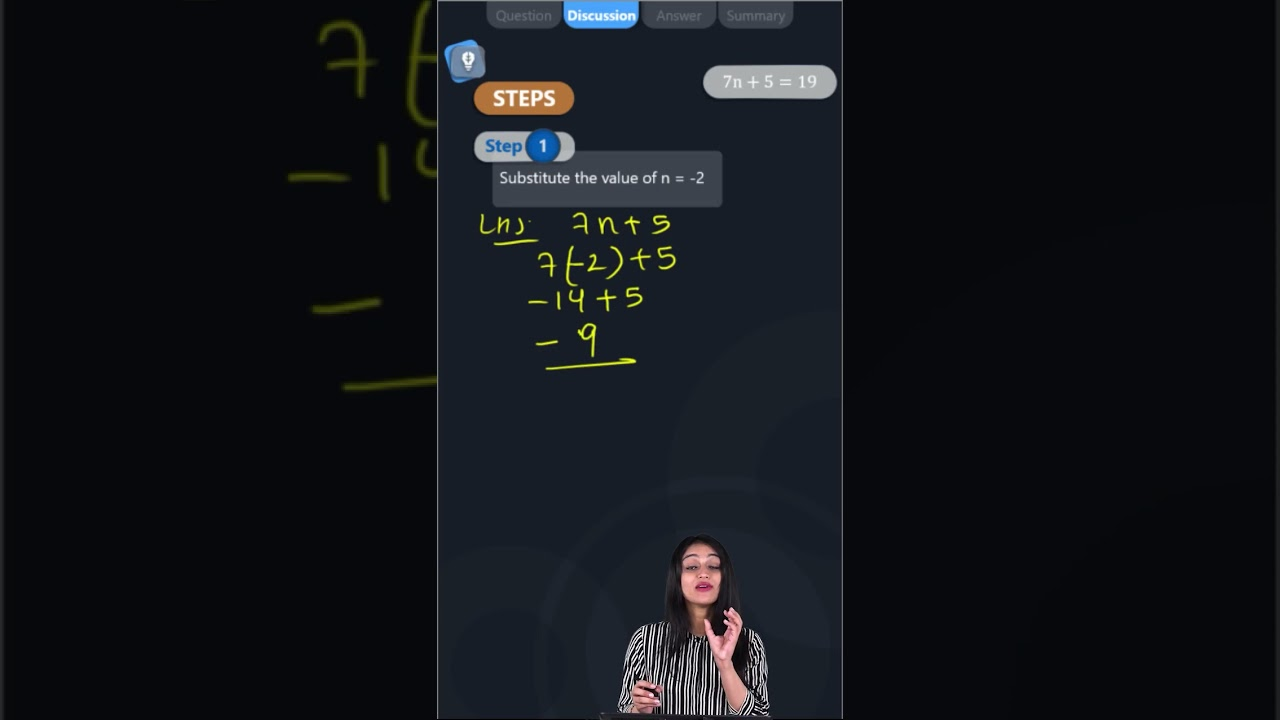Check whether the value given in the brackets is a solution to the given equation or not: 7n + 5 = 19 (n = - 2)Given:7n + 5 = 19 (n = – 2)

Consider LHS of the equation

LHS = 7n + 5

By substituting the value of n = -2

Then,

LHS = 7n + 5

LHS = (7 × (-2)) + 5

LHS = – 14 + 5

LHS = – 9

By comparing LHS and RHS

-9 ≠ 19

LHS ≠ RHS

Hence, the value of n = -2 is not a solution to the given equation 7n + 5 = 19.(0)(1)